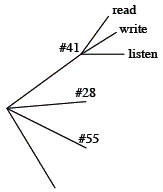### Home > INT2 > Chapter 4 > Lesson 4.2.1 > Problem4-66

4-66.To get home, Renae can take one of four buses: #41, #28, #55, or #81. Once she is on a bus, she will randomly select one of the following activities: listening to her MP3 player, writing a letter, or reading a book.

1. Create a tree diagram to show all of the different travel options that Renae could take.

Here is a start to the tree diagram:2. Use your tree diagram to determine the following probabilities:

1. $P$(Renae takes an odd-numbered bus)

2. $P$(Renae does not write a letter)

3. $P$(Renae catches the #28 bus and then reads a book)

4. $P$(Renae does not read on the way home)

Assuming all outcomes are equally likely:

$\text{probability}=\frac{\text{number of desired outcomes}}{\text{number of possible outcomes}}$

Find the numerator by counting from your tree diagram.

$\frac{8}{12}$

3. Does her activity depend on which bus she takes? Explain why or why not.

Look at your tree diagram. Do the possible activities change depending on which bus she takes?